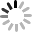工作时间

联系方式

# 标准曲线的绘制方法

ELISA的标准曲线一般使用专门的曲线拟合工具，如：Curve Expert 1.3

1.  启动“Curve  Expert1.3”

2．X轴输入所对应的浓度值，Y轴输入标准品的OD值，如图：

Normally people draw ELISA standard curve by using specialized tools for curve fitting, such as: Curve Expert 1.3

Below is "Curve Exert1.3" software, for example, draw the ELISA standard curve as follows:

2. X-axis: input values corresponding to the concentration, Y-axis: input the OD values of standard, as shown:3．单击[运行]按钮，出现如下对话框

Click [Run] button, the following dialog box appears4．单击[ok]按钮，出现如下两个对话框，关闭下面一个对话框

Click [ok] button, the following two dialog boxes appear, then close the dialog box below5. 在对话框的右上角出现一些曲线的名称，从“1”开始依次点击曲线名称，在右下角会出现相应拟合的曲线。

A list of some curves appears at the upper right corner of the dialog box, from "1" Start to click the name of curves, then the corresponding fitting curve will appear in the lower right corner.6. 根据拟合的曲线选取ELISA拟合度最佳的曲线双击，出现如下对话框：

Double click the best fitting curve for ELISA test, the dialog box appears as follows:

Note: select curve equation with the best coefficient (the "r" value) for computing. In the following dialog box, the "r" value at the upper right corner (close to 1 means the better fitting)7．按[Ctrl]键+[L]键，出现如下对话框：

Press [Ctrl] key + [L] key, the following dialog box appears:8．输入标准的OD值，单击[Calculate]按纽，即可得到待测蛋白的实际含量。（标本稀释了N倍，运算出的数值应再成以N）。

Enter the standard OD values, click [Calculate] button, you can get the actual content of the target protein. (N-fold diluted samples, calculate the value should be multiplied by N).

9. 如想得到ELISA拟合曲线的方程，可在步骤6的对话框空白处右击，选择”Information”

If you would like to have the curve fitting equation of ELISA, right-click blank space of the dialog box in step 6, choose "Information"

10. 得到如下对话框：点击“Copy”

Get the following dialog box: Click "Copy''You can paste in the location you want to obtain the data as follows:

MMF Model: y=(a*b+c*x^d)/(b+x^d)

Coefficient Data:

a = 7.01345230536E-002

b = 2.02844521674E+003

c = 2.36893396063E+001

d = 9.31891501118E-001联系方式